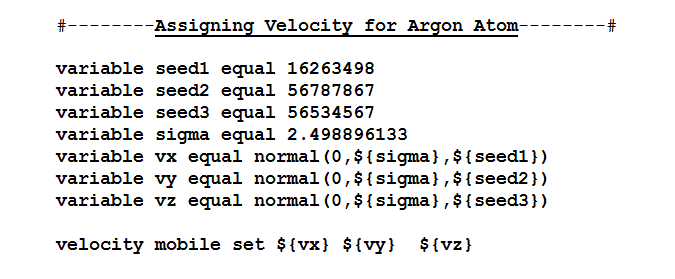# How to assign abs() function to the variable command?

Hi All,

I would like to assign only negative velocities to vz velocity component as shown below. I just could not figure out how to use abs () function to do so. I have tried different versions but they all gave error.
Attached one is the code to which I would like to assign (-) vz velocity component. I would appreciate if someone can help with this issue or send me a link which explains it.

Thanks,
RoseHi All,

I would like to assign only negative velocities to vz velocity component as shown below. I just could not figure out how to use abs () function to do so. I have tried different versions but they all gave error.
Attached one is the code to which I would like to assign (-) vz velocity component. I would appreciate if someone can help with this issue or send me a link which explains it.

why don't you let us first know what you tried and what the error
messages are? this way we can actually see where your mistake is.

the abs() function has to be used just as you would expect:
abs(sin(\$x)) will give you the absolute of the sine of the value of
\$x.

(pseudo) random number generators in the variable command
documentation.

axel.

Hi Axel,
Thanks for your response. Below is what I have tried:

variable vx equal normal (0, \{sigma\}, {seed1})
variable vy equal normal (0, \{sigma\}, {seed2})
variable vz equal normal (0, \{sigma\}, {seed3})

velocity mobile set \{vx\} {vy} -abs( \${vz})

I was hoping to get "negative velocity components for vz" everytime I run the simulation with different seed numbers.

Here is the error I got: Expected floating point parameter in input script or data file. (..//velocity.cpp:375)

Thanks,
Rose

Hi Axel,
Thanks for your response. Below is what I have tried:

variable vx equal normal (0, \{sigma\}, {seed1})
variable vy equal normal (0, \{sigma\}, {seed2})
variable vz equal normal (0, \{sigma\}, {seed3})

velocity mobile set \{vx\} {vy} -abs( \${vz})

I was hoping to get "negative velocity components for vz" everytime I run the simulation with different seed numbers.

Here is the error I got: Expected floating point parameter in input script or data file. (..//velocity.cpp:375)

yes. and deservedly so. what you do is wrong. the velocity command
expects a number and gets "-abs(" instead. that doesn't work. you
cannot just apply variable expressions anywhere in your input. they
can only be used in variable definitions.

at the very least you have to use an immediate variable using the
\(\-abs\({vz})) syntax. this is all explained in the documentation for
the variable command.

axel.